Courses

# Test: Heat Transfer By Natural Convection - 2

## 8 Questions MCQ Test Topicwise Question Bank for Mechanical Engineering | Test: Heat Transfer By Natural Convection - 2

Description
This mock test of Test: Heat Transfer By Natural Convection - 2 for Chemical Engineering helps you for every Chemical Engineering entrance exam. This contains 8 Multiple Choice Questions for Chemical Engineering Test: Heat Transfer By Natural Convection - 2 (mcq) to study with solutions a complete question bank. The solved questions answers in this Test: Heat Transfer By Natural Convection - 2 quiz give you a good mix of easy questions and tough questions. Chemical Engineering students definitely take this Test: Heat Transfer By Natural Convection - 2 exercise for a better result in the exam. You can find other Test: Heat Transfer By Natural Convection - 2 extra questions, long questions & short questions for Chemical Engineering on EduRev as well by searching above.
QUESTION: 1

### The convective heat transfer coefficient from a hot cylindrical surface exposed to still air varies in accordance with  Where ΔT is the temperature difference between the hot surface and surrounding ambient air.

Solution: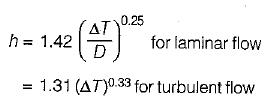QUESTION: 2

### The nusselt number of convective heat transfer between a horizontal tube and water surrounding it is prescribed by the relation Nu = 0.52 (Gr Pr)0.25 for a 5 cm diameter tube, the heat transfer coefficient is 6000 W/m2-K. Subsequently, the tube is replaced by one with 20 cm diameter tube. If the temperature and surface of the fluid remain same, the heat transfer coefficient will change to

Solution: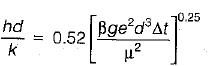∴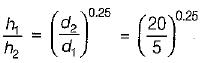∴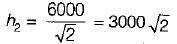= 4242W/m2-K

QUESTION: 3

### In convection heat transfer one of the significant dimensionless number is

Solution:
QUESTION: 4

Nusselt number in case of free convection is the function of

Solution:

Nu = f(Gr, Pr)
Rayleigh number = Gr x Pr.

QUESTION: 5

When there is flow of fluid over a flat plate of length L the average heat transfer coefficient is given by (Nux = Local nusselt number)

Solution: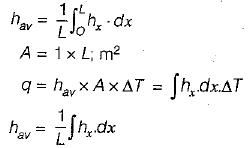QUESTION: 6

The Grashoff number is

Solution:
QUESTION: 7

The temperature profile for heat transfer from one fluid to another separated by a solid wall is

Solution:
QUESTION: 8

Match List-I with List-II and select the correct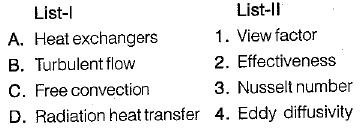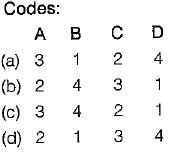Solution: